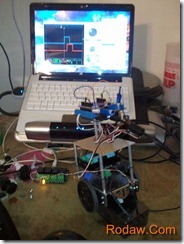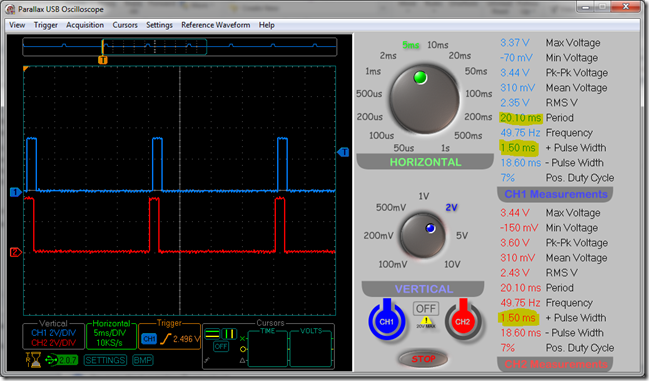Categories

# Dottie; BoeDuinoBot.Net

Meet Dottie (Doing Other Technology That Is Extensible) my BoeDuinoBot.Net Robot built on a Parallax Boe-Bot (parts kit) with a netduinoPlus (a netduino is in the mail somewhere…) rather than a basic stamp. Note the Parallax Li-ion Power Pack Mounted under my BoeDuinoBot.Net RCB (Robot Controller Board).

I’m planning to control the Boe-Bot from Arduino and Netduino. I’m building a PCB shield to support both platforms. Check out my effort to move from concept, prototype to PCB board. I learned about Fritzing and etched my own PCB at home with a toner transfer method using an easy process using laser printer and clothes Iron.

I’ll be blogging further on this project as I drill the PCB and install it on Dottie, add sensors and continue refactoring BoeDuinoBot.Net.

Dottie got off to a little bit of a wobbly start in this very first prototype of the basic hardware platform. Uncentered servos and a high center of gravity will be fixed right away. Read on.Running this code on Dottie results in the following wave form.

`using SecretLabs.NETMF.Hardware;`
`using SecretLabs.NETMF.Hardware.NetduinoPlus;`
` `
`namespace CenterServos`
`{`
`    public class Program`
`    {`
`        public static void Main()`
`        {`
`            PWM leftServo = new PWM(Pins.GPIO_PIN_D5);`
`            PWM rightServo = new PWM(Pins.GPIO_PIN_D6);`
` `
`            leftServo.SetPulse(20000, 1500);`
`            rightServo.SetPulse(20000, 1500);`
`        }`
`    }`
`}`

Very solid 1.50ms pulse for center and the frequency of 49.75 reciprocal is pretty darn close to 20ms keep alive.

The continuous motion servos were no where near centered. Continuous motion servos should be still with no rotation when fed a 1.5ms signal. I adjusted a set screw on the servos to stop there motion while being fed this signal generated from the previous code.Centering the servos and lowering the center of gravity has improved Dottie’s stability.

Here is the program I ran for the above video. Not sure I want to share the boeNetBot class yet until I clean it up a bit. It’s a mess right now.

Hmm… The code insert plugin expanded all my carefully collapsed #regions I had created to increase readability. The commented out code might be instructive. Some of the test I ran in development of Dottie.

Note: This is pretty early code. I’ve been refactoring quite a bit. I”ll have another article once I’ve cleaned up the code and perhaps added a sensor or two.

`using System;`
`using System.Threading;`
`using Microsoft.SPOT.Hardware;`
`using SecretLabs.NETMF.Hardware.NetduinoPlus;`
`using Servo_API;    // I enhanced this code from Chris Seto for continuous motion servos:`
`                    // http://forums.netduino.com/index.php?/topic/160-netduino-servo-class/`
` `
`// going to look at `
`// http://netduinohelpers.codeplex.com/SourceControl/changeset/view/882d05abd90d`
`// when I start the next round of refactoring. `
` `
`namespace boeNetBot`
`{`
`    public class Program`
`    {`
`        //static int tPause = 10;`
` `
`        static Servo leftServo = new Servo(Pins.GPIO_PIN_D5);       // PWM pins`
`        static Servo rightServo = new Servo(Pins.GPIO_PIN_D6);`
` `
`        static BoeNetBot boeDot = new BoeNetBot(leftServo, rightServo);`
` `
`        public static void Main()`
`        {`
`            // Use the button on the protoshield for a ALL Stop! panic button.`
`            InterruptPort stopButton =      `
`                new InterruptPort(Pins.GPIO_PIN_D13, true, `
`                    Port.ResistorMode.PullUp, Port.InterruptMode.InterruptEdgeLevelHigh);`
` `
`            stopButton.OnInterrupt += new NativeEventHandler(stopButton_OnInterrupt);`
` `
`            #region test code`
`            //for (int a = 0; a < 3; a++)`
`            //{`
`            //    leftServo.Degree = speed;`
`            //    rightServo.Degree = speed;`
`            //    Thread.Sleep(3000);`
`            //    leftServo.disengage();`
`            //    rightServo.disengage();`
`            //    Thread.Sleep(1500);`
`            //} `
`            #endregion`
` `
`            rightServo.inverted = true;`
`            int l = 1250;`
`            int r = 1750;`
`            leftServo.setRange(l, r);`
`            rightServo.setRange(l, r);`
`            `
`            boeDot.speed = 25;`
` `
`            while (true)`
`            {`
`                boeDot.direction = Directions.forward;`
`                ps(2);`
`                boeDot.direction = Directions.left;`
`                pm(350);`
`                boeDot.direction = Directions.forward;`
`                ps(2);`
`                boeDot.direction = Directions.left;`
`                pm(350);`
`            }`
` `
`            #region servo ramping test code`
`            //while (true)`
`            //{`
`            //    boeDot.direction = Directions.forward;`
`            //    for (int i = 100; i > 0; i--)`
`            //    {`
`            //        boeDot.speed = i;`
` `
`            //    }`
`            //    for (int i = 0; i < 100; i++)`
`            //    {`
`            //        boeDot.speed = i;`
`            //    }`
`            //    boeDot.direction = Directions.stop;`
`            //    //Thread.Sleep(1500);`
` `
`            //} `
`            #endregion`
` `
`            #region simple test`
`            //while (true)`
`            //{`
`            //    for (int l = 0, r = 0; l <= 180; l++, r++)`
`            //    {`
`            //        leftServo.Degree = l;`
`            //        rightServo.Degree = r;`
`            //        Thread.Sleep(tPause);`
`            //    }`
` `
` `
` `
`            //    for (int l = 180, r = 180; l >= 0; l--, r--)`
`            //    {`
`            //        leftServo.Degree = l;`
`            //        rightServo.Degree = r;`
`            //        Thread.Sleep(tPause);`
`            //    }`
`            //}`
`            #endregion`
`        }`
` `
`        static void stopButton_OnInterrupt(uint data1, uint data2, DateTime time)`
`        {`
` `
`            boeDot.direction = Directions.stop;`
`            boeDot.Dispose();`
`        }`
` `
`        /// <summary>`
`        /// ps pause in Seconds.`
`        /// </summary>`
`        /// <param name="i"></param>`
`        static void ps(double  i)`
`        {`
`            Thread.Sleep((int)i*1000);`
`        }`
` `
`        /// <summary>`
`        /// pause in ms. 'cause I'm tired of writing out Thread.Sleep`
`        /// </summary>`
`        /// <param name="i"></param>`
`        static void pm(int i)`
`        {`
`            Thread.Sleep(i);`
`        }`
`    }`
`}`

Now that I have the basic physical robot created and a basic layout of the boeDuinoBot.Net class working I’ll be adding some sensors to help Dottie negotiate on her own a bit. I have some Infrared sensors on order.

Stay tuned.